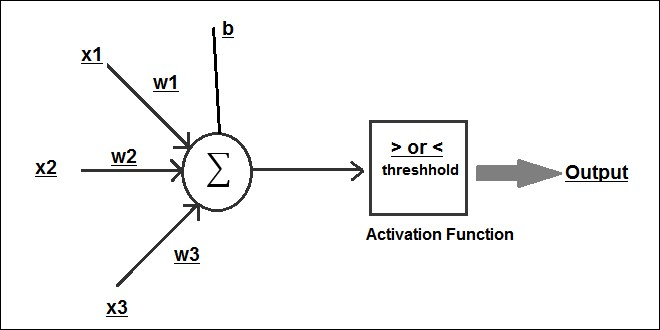•#### Big Data Analytics with Java#### Overview of this book

This book covers case studies such as sentiment analysis on a tweet dataset, recommendations on a movielens dataset, customer segmentation on an ecommerce dataset, and graph analysis on actual flights dataset. This book is an end-to-end guide to implement analytics on big data with Java. Java is the de facto language for major big data environments, including Hadoop. This book will teach you how to perform analytics on big data with production-friendly Java. This book basically divided into two sections. The first part is an introduction that will help the readers get acquainted with big data environments, whereas the second part will contain a hardcore discussion on all the concepts in analytics on big data. It will take you from data analysis and data visualization to the core concepts and advantages of machine learning, real-life usage of regression and classification using Naïve Bayes, a deep discussion on the concepts of clustering,and a review of simple neural networks on big data using deepLearning4j or plain Java Spark code. This book is a must-have book for Java developers who want to start learning big data analytics and want to use it in the real world.
Big Data Analytics with JavaCreditswww.PacktPub.comCustomer FeedbackPrefaceFree Chapter
Big Data Analytics with JavaFirst Steps in Data AnalysisData VisualizationBasics of Machine LearningRegression on Big DataNaive Bayes and Sentiment AnalysisDecision TreesEnsembling on Big DataRecommendation SystemsClustering and Customer Segmentation on Big DataMassive Graphs on Big DataReal-Time Analytics on Big DataDeep Learning Using Big DataIndex## Perceptron

A perceptron is a type of artificial neuron that is mathematical and programmatic. It takes in many inputs and applies weights to them based on the importance of the inputs, and then adds a bias before using this mathematical approach to figure out a result. This result from the perceptron is then fed to a machine learning algorithm, such as logistic regression. We call this algorithm as an activation function, which is then is used to predict the final result of the outcome.

The perceptron is depicted as follows:As you can see in the previous image, a perceptron depicts an artificial neuron that takes in various inputs in binary form and multiplies them with a weight, w. The weight is calculated based on the importance of the input. A bias value is also added, along with the weights. Now, the entire combination is summed up by the perceptron. Finally, the summed-up output is tested against a threshold value, and we call this as an Activation Function. If the value is above a threshold...